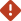# Investment Income Calculator

Enter values in any 2 of the fields below to estimate the yield, potential income, or amount for a hypothetical investment. Then click Calculate your results.
Type in estimated yield percentageError: Enter values in any 2 of the fields above to estimate the yield, potential income, or amount for a hypothetical investment. Then click Calculate your results.Error: Enter a yield greater than 0.0 and less than or equal to 20.0.
Type in dollar amountError: Enter values in any 2 of the fields above to estimate the yield, potential income, or amount for a hypothetical investment. Then click Calculate your results.Error: Enter an investment amount between \$1,000 and \$999,999,999 .
Type in desired income amountError: Enter values in any 2 of the fields above to estimate the yield, potential income, or amount for a hypothetical investment. Then click Calculate your results.Error: Enter a yearly income less than \$200,000,003.Error: Enter a monthly income less than \$16,666,667.Error: Fill only 2 input fields. Remove 1 entry and click Calculate your resultsError: We'll calculate results using your figure with only the first 2 numbers to the right of the decimal point.

See a list of Vanguard funds to find a fund that meets your needs.

Note: Factors such as bond maturity and income tax bracket should be considered when analyzing calculation results.
The calculations displayed do not represent an investment in any particular fund and are not an indication of future results that may be achieved. Yields can change on a daily basis, and the amount of income can vary significantly with changing interest rates. Higher rates of return often involve a higher level of risk.